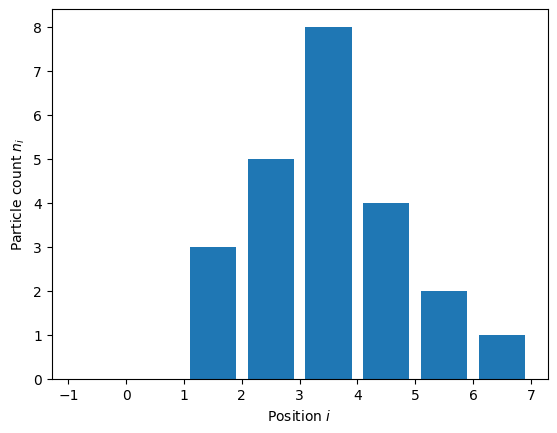# 5.3 Classroom exercise: energy calculation#

Estimated time for this notebook: 30 minutes

## Diffusion model in 1D#

Description: A one-dimensional diffusion model. (Could be a gas of particles, or a bunch of crowded people in a corridor, or animals in a valley habitat…)

• Agents are on a 1d axis

• Agents do not want to be where there are other agents

• This is represented as an ‘energy’: the higher the energy, the more unhappy the agents.

Implementation:

• Given a vector $$n$$ of positive integers, and of arbitrary length

• Compute the energy, $$E(n) = \sum_i n_i(n_i - 1)$$

• Later, we will have the likelyhood of an agent moving depend on the change in energy.

%matplotlib inline
import numpy as np
from matplotlib import pyplot as plt

density = np.array([0, 0, 3, 5, 8, 4, 2, 1])
fig, ax = plt.subplots()
ax.bar(np.arange(len(density)) - 0.5, density)
ax.xrange = [-0.5, len(density) - 0.5]
ax.set_ylabel("Particle count $n_i$")
ax.set_xlabel("Position $i$")

Text(0.5, 0, 'Position $i$')Here, the total energy due to position 2 is $$3 (3-1)=6$$, and due to column 7 is $$1 (1-1)=0$$. We need to sum these to get the total energy.

## Starting point#

Create a Python module:

%%bash
rm -rf diffusion
mkdir diffusion
touch diffusion/__init__.py


Windows: You will need to run the following instead

%%cmd
rmdir /s diffusion
mkdir diffusion
type nul > diffusion/__init__.py


NB. If you are using the Windows command prompt, you will also have to replace all subsequent %%bash directives with %%cmd

• Implementation file: diffusion_model.py

%%writefile diffusion/model.py
def energy(density, coeff=1.0):
"""Energy associated with the diffusion model

Parameters
----------

density: array of positive integers
Number of particles at each position i in the array
coeff: float
Diffusion coefficient.
"""
# implementation goes here

Writing diffusion/model.py

• Testing file: test_diffusion_model.py

%%writefile diffusion/test_model.py
from .model import energy

def test_energy():
pass
# Test something

Writing diffusion/test_model.py


Invoke the tests:

%%bash
cd diffusion
pytest

============================= test session starts ==============================

platform linux -- Python 3.8.17, pytest-7.4.0, pluggy-1.2.0

rootdir: /home/runner/work/rse-course/rse-course/module05_testing_your_code/diffusion

plugins: pylama-8.4.1, cov-4.1.0, anyio-3.7.1

collected 1 item



test_model.py .                                                          [100%]



============================== 1 passed in 0.01s ===============================


Now, write your code (in model.py), and tests (in test_model.py), testing as you do.

## Solution#

Don’t look until after you’ve tried!

In the spirit of test-driven development let’s first consider our tests.

%%writefile diffusion/test_model.py
"""Unit tests for a diffusion model."""

from pytest import raises
from .model import energy

def test_energy_fails_on_non_integer_density():
with raises(TypeError):
energy([1.0, 2, 3])

def test_energy_fails_on_negative_density():
with raises(ValueError):
energy([-1, 2, 3])

def test_energy_fails_ndimensional_density():
with raises(ValueError):
energy([[1, 2, 3], [3, 4, 5]])

def test_zero_energy_cases():
# Zero energy at zero density
densities = [[], , [0, 0, 0]]
for density in densities:
assert energy(density) == 0

def test_derivative():
from numpy.random import randint

# Loop over vectors of different sizes (but not empty)
for vector_size in randint(1, 1000, size=30):

# Create random density of size N
density = randint(50, size=vector_size)

# will do derivative at this index
element_index = randint(vector_size)

# modified densities
density_plus_one = density.copy()
density_plus_one[element_index] += 1

# Compute and check result
# d(n^2-1)/dn = 2n
expected = 2.0 * density[element_index] if density[element_index] > 0 else 0
actual = energy(density_plus_one) - energy(density)
assert expected == actual

def test_derivative_no_self_energy():
"""If particle is alone, then its participation to energy is zero."""
from numpy import array

density = array([1, 0, 1, 10, 15, 0])
density_plus_one = density.copy()
density += 1

expected = 0
actual = energy(density_plus_one) - energy(density)
assert expected == actual

Overwriting diffusion/test_model.py


Now let’s write an implementation that passes the tests.

%%writefile diffusion/model.py
"""Simplistic 1-dimensional diffusion model."""
from numpy import array, any, sum

def energy(density):
"""Energy associated with the diffusion model
:Parameters:
density: array of positive integers
Number of particles at each position i in the array/geometry
"""

# Make sure input is an numpy array
density = array(density)

# ...of the right kind (integer). Unless it is zero length,
#    in which case type does not matter.

if density.dtype.kind != "i" and len(density) > 0:
raise TypeError("Density should be a array of *integers*.")
# and the right values (positive or null)
if any(density < 0):
raise ValueError("Density should be an array of *positive* integers.")
if density.ndim != 1:
raise ValueError(
"Density should be an a *1-dimensional*" + "array of positive integers."
)

return sum(density * (density - 1))

Overwriting diffusion/model.py

%%bash
cd diffusion
pytest

============================= test session starts ==============================

platform linux -- Python 3.8.17, pytest-7.4.0, pluggy-1.2.0

rootdir: /home/runner/work/rse-course/rse-course/module05_testing_your_code/diffusion

plugins: pylama-8.4.1, cov-4.1.0, anyio-3.7.1

collected 6 items



test_model.py ......                                                     [100%]



============================== 6 passed in 0.11s ===============================


## Coverage#

With pytest, you can use the “pytest-cov” plugin to measure test coverage

%%bash
cd diffusion
pytest --cov

============================= test session starts ==============================

platform linux -- Python 3.8.17, pytest-7.4.0, pluggy-1.2.0

rootdir: /home/runner/work/rse-course/rse-course/module05_testing_your_code/diffusion

plugins: pylama-8.4.1, cov-4.1.0, anyio-3.7.1

collected 6 items



test_model.py ......                                                     [100%]



---------- coverage: platform linux, python 3.8.17-final-0 -----------

Name            Stmts   Miss  Cover

-----------------------------------

__init__.py         0      0   100%

model.py           10      0   100%

test_model.py      33      0   100%

-----------------------------------

TOTAL              43      0   100%





============================== 6 passed in 0.19s ===============================


Or an html report:

%%bash
#%%cmd (windows)
cd diffusion
pytest --cov --cov-report html

============================= test session starts ==============================

platform linux -- Python 3.8.17, pytest-7.4.0, pluggy-1.2.0

rootdir: /home/runner/work/rse-course/rse-course/module05_testing_your_code/diffusion

plugins: pylama-8.4.1, cov-4.1.0, anyio-3.7.1

collected 6 items



test_model.py ......                                                     [100%]



---------- coverage: platform linux, python 3.8.17-final-0 -----------

Coverage HTML written to dir htmlcov





============================== 6 passed in 0.20s ===============================


The HTML coverage results will be in diffusion/htmlcov/index.html